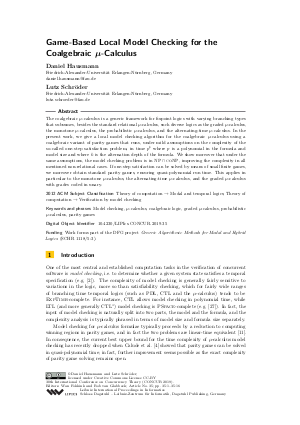Document# Game-Based Local Model Checking for the Coalgebraic mu-Calculus

### Authors Daniel Hausmann, Lutz Schröder## File

LIPIcs.CONCUR.2019.35.pdf
• Filesize: 0.51 MB
• 16 pages

## Cite As

Daniel Hausmann and Lutz Schröder. Game-Based Local Model Checking for the Coalgebraic mu-Calculus. In 30th International Conference on Concurrency Theory (CONCUR 2019). Leibniz International Proceedings in Informatics (LIPIcs), Volume 140, pp. 35:1-35:16, Schloss Dagstuhl - Leibniz-Zentrum für Informatik (2019)
https://doi.org/10.4230/LIPIcs.CONCUR.2019.35

## Abstract

The coalgebraic mu-calculus is a generic framework for fixpoint logics with varying branching types that subsumes, besides the standard relational mu-calculus, such diverse logics as the graded mu-calculus, the monotone mu-calculus, the probabilistic mu-calculus, and the alternating-time mu-calculus. In the present work, we give a local model checking algorithm for the coalgebraic mu-calculus using a coalgebraic variant of parity games that runs, under mild assumptions on the complexity of the so-called one-step satisfaction problem, in time p^k where p is a polynomial in the formula and model size and where k is the alternation depth of the formula. We show moreover that under the same assumptions, the model checking problem is in both NP and coNP, improving the complexity in all mentioned non-relational cases. If one-step satisfaction can be solved by means of small finite games, we moreover obtain standard parity games, ensuring quasi-polynomial run time. This applies in particular to the monotone mu-calculus, the alternating-time mu-calculus, and the graded mu-calculus with grades coded in unary.

## Subject Classification

##### ACM Subject Classification
• Theory of computation → Modal and temporal logics
• Theory of computation → Verification by model checking
##### Keywords
• Model checking
• mu-calculus
• coalgebraic logic
• probabilistic mu-calculus
• parity games

## Metrics

• Access Statistics
• Total Accesses (updated on a weekly basis)
0